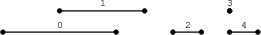Your browser (Unknown 0) is no longer supported. Some parts of the website may not work correctly. Please update your browser.

#### MaxNonoverlappingSegments

Find a maximal set of non-overlapping segments.

Located on a line are N segments, numbered from 0 to N − 1, whose positions are given in arrays A and B. For each I (0 ≤ I < N) the position of segment I is from A[I] to B[I] (inclusive). The segments are sorted by their ends, which means that B[K] ≤ B[K + 1] for K such that 0 ≤ K < N − 1.

Two segments I and J, such that I ≠ J, are overlapping if they share at least one common point. In other words, A[I] ≤ A[J] ≤ B[I] or A[J] ≤ A[I] ≤ B[J].

We say that the set of segments is non-overlapping if it contains no two overlapping segments. The goal is to find the size of a non-overlapping set containing the maximal number of segments.

For example, consider arrays A, B such that:

A = 1 B = 5 A = 3 B = 6 A = 7 B = 8 A = 9 B = 9 A = 9 B = 10

The segments are shown in the figure below.The size of a non-overlapping set containing a maximal number of segments is 3. For example, possible sets are {0, 2, 3}, {0, 2, 4}, {1, 2, 3} or {1, 2, 4}. There is no non-overlapping set with four segments.

Write a function:

class Solution { public int solution(int[] A, int[] B); }

that, given two arrays A and B consisting of N integers, returns the size of a non-overlapping set containing a maximal number of segments.

For example, given arrays A, B shown above, the function should return 3, as explained above.

Write an efficient algorithm for the following assumptions:

• N is an integer within the range [0..30,000];
• each element of arrays A and B is an integer within the range [0..1,000,000,000];
• A[I] ≤ B[I], for each I (0 ≤ I < N);
• B[K] ≤ B[K + 1], for each K (0 ≤ K < N − 1).